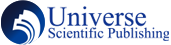Journals Account Articles Publisher Editorial Team Announcement

### Operational Matrix Basic Spline Wavelets of Derivative for linear Optimal Control Problem

Maha Delphi, Suha SHIHAB

#### Abstract

The main importance goal in this paper is studying the interesting properties of basic spline wavelets functions (BSWFs) and derived some new basic formulations of them. The important operational matrix is devoted in two ways, the first one is the derivative of BSWFs in terms of the lower order of  BSWFs  while the second is the derivative of BSWFs in terms of the same order of BSWFs.  The expression formula for the operational matrix  is determined for different orders. In addition an useful formulas concerning the power function and BSMSFs are also presented. The polynomials and wavelets expansions together with operational matrices can be employed to solve problems in applied science and other fields of approximation theory. In this work, two optimal control problem are tested with the aid of operational matrix of derivative for BSWFs with satisfactory results.

#### Keywords

Basic spline wavelets; Operational matrix; Linear optimal control problem

PDF

#### References

S. A. Yousefi, B-polynomial multiwavelets approach for the solution of Abel's integral equation, International Journal of Computer Mathematics, 87 (2) (2013) 310-316.

A. K. Nasab, A. Kılıçman, E. Babolian, Wavelet analysis method for solving linear and nonlinear singular boundary value problems, Applied Mathematical Modelling, 37 (8) (2013) 5876-5886.

I. Hashim, M. Alshbool, Solving directly third-order ODEs using operational matrices of Bernstein polynomials method with applications to fluid flow equations, Journal of King Saud University-Science, (2018) in press.

K. Parand, S. A. Kaviani, Application of the exact operational matrices based on the Bernstein polynomials, Journal of Mathematics and Computer Science, 6 (2013) 36-59.

T. Ismaeelpour, A.A. Hemmat, H. Saeedi, B-spline operational matrix of fractional integration, Optik. 130 (2017) 291–305.

Shihab S., Sarhan M., New Operational Matrices of Shifted Fourth Chebyshev wavelets, Elixir International Journal-Applied Mathematics, 69 (1) (2014) 23239-23244.

H. Hassani, J.A. Tenreiro Machado, E. Narathiwat, Generalized shifted Chebyshev polynomials for fractional optimal control problems, Commun. Nonlinear Sci. Numer. Simul. 75 (2019) 50–61.

I. Malmir, Novel Chebyshev wavelets algorithms for optimal control and analysis of general linear delay models, Appl. Math. Model. 69 (2019) 621–647.

A. Lotfi, S.A. Yousefi, M. Dehghan, Numerical solution of a class of fractional optimal control problems via the Legendre orthonormal basis combined with the operational matrix and the Gauss quadrature rule, J. Comput. Appl. Math. 250 (2013) 143–160.

The Taylor wavelets method for solving the initial and boundary value problems of Bratu-type equations, Appl. Numer. Math. 128 (2018) 205–216.

Z. Foroozandeh, M. Shamsi, Solution of nonlinear optimal control problems by the interpolating scaling functions, Acta Astronaut. 72 (2012) 21–26.

Shihab, H. R. Al-Rubaie, an Approximate solution of some continuous time Linear-Quadratic optimal control problem via Generalized Laguerre Polynomial, Journal of Pure and Applied Sciences, 22 (1) (2010) 85-97.

J. A. Eleiwy, S. N. Shihab, Chebyshev Polynomials and Spectral Method for Optimal Control Problem, Engineering and Technology Journal, 27 (14) (2009) 2642-2652.

S. N. Shihab, Mohammed Abdelhadi Sarhan, Convergence analysis of shifted fourth kind Chebyshev wavelets, IOSR Journal of Mathematics, 10 (2), 54-58.

Suha Al-Rawi, NUMERICAL SOLUTION OF INTEGRAL EQUATIONS USING TAYLOR SERIES, Journal of the College of Education, 5, 51-60.

Suha Al-Rawi, F. A. Al-Heety, S. S. Hasan, A new Computational Method for Optimal Control Problem with B-spline Polynomials, Engineering and Technology Journal 28 (18), 5711-5718, 2010.

S. N. Shihab, T. N. Naif, On the orthonormal Bernstein polynomial of order eight, open Science Journal of Mathematics and Application, 2 (2) (2014) 15-19.

Maha Delphi, Suha SHIHAB, Operational Matrix Basic Spline Wavelets of Derivative for linear Optimal Control Problem, Electronics Science Technology and Application 6 (2), 18-24.

Suha Al-Rawi, On the Solution of Certain Fractional Integral Equations, Kirkuk University Journal for Scientific Studies 1 (2), 125-136 (2006).

DOI: http://dx.doi.org/10.18686/esta.v6i2.91

### Refbacks

• There are currently no refbacks.